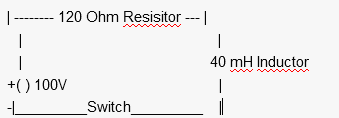# Current through an inductor after a switch closes

• David Donald

## Homework StatementLet's say I have a circuit such as the one above, and let's say the circuit has been
open for a long time.

How would I find the current through the inductor, the instant after closing the switch?

and the voltage potential across the 40 mh inductor?

## Homework Equations

I(t) = E/R ( 1 - e^(-R/L*t) )

## The Attempt at a Solution

I'm a bit lost would the current just be the voltage/resistor = 100/120 = .83333 amps??

how would I find the current right after the switch closes?
and then how would I use that to find the voltage across the 30 mH inductor?

Could someone give me an explanation on how to find the current through the inductor and voltage potential, I'm quite lost and confused : /

would the current just be the voltage/resistor = 100/120 = .83333 amps??
No. Put t=0 in this equation.
I(t) = E/R ( 1 - e^(-R/L*t) )

So the current would just then be 0 ? I(t) = E/R ( 1 - 1) (0) = (0)

So the current would just then be 0 ?
Yes. Inductor acts as an open switch at this instant. What will be the voltage across this open switch?

Yes. Inductor acts as an open switch at this instant. What will be the voltage across this open switch?

It's 100 volts, I'm not going to bother writing all the work out here since I just derived it on paper.
My confusion just stemmed from the behavior of the inductor itself...